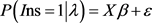﻿ 劳动力工伤保险参保差异分析

# 劳动力工伤保险参保差异分析Analysis on the Difference of Industrial Injury Insurance

Abstract: Based on CLDS2016 data, this paper uses the Blinder-Oaxaca decomposition method of nonlinear model to study the differences and reasons of urban and rural labor force and male and female labor force participating in industrial injury insurance. The results show that there are significant differences in the participation of industrial injury insurance between urban and rural workers, and there is household registration discrimination against rural labor force, which accounts for 43.1% of the total difference between urban and rural areas. There are also significant differences in the participation of workers of different genders in industrial injury insurance, and the degree of gender discrimination is greater than that of household registration.

1. 引言

2. 文献综述

3. 数据来源及变量说明Table 1. Variable descriptive statisticsTable 2. Number of units and industrial injury insurance participation rate

4. 模型及差异分解

4.1. Blinder-Oaxaca分解方法

Blinder-Oaxaca分解方法是Blinder (1973)和Oaxaca (1973)提出的反事实分解技术，该技术被广泛用于研究两个群体间的平均结果差异 。该项技术在很多劳动力市场和歧视的文献中应用，同时该方法在可其他领域应用，例如，本文研究了城乡劳动力的差异。Oaxaca (1973)的提出有两个新的研究目的，一个是研究度量性别歧视的严重程度；另一个目的是研究分析衡量影响不同性别平均工资收入差距的各种因素的相对性和重要性。Oaxaca (1973)  对歧视的术语定义式为：

$D=\frac{{W}_{c}/{W}_{t}-{\left({W}_{c}/{W}_{t}\right)}^{0}}{\left({W}_{c}/{W}_{t}\right)}$ (4.1)

$\begin{array}{l}\mathrm{ln}\stackrel{¯}{{W}_{c}}-\mathrm{ln}\stackrel{¯}{{W}_{t}}=\left(\stackrel{¯}{{X}_{c}}-\stackrel{¯}{{X}_{t}}\right){\stackrel{^}{\beta }}_{t}+\stackrel{¯}{{X}_{c}}\left({\stackrel{^}{\beta }}_{c}-{\stackrel{^}{\beta }}_{t}\right)\\ \mathrm{ln}\stackrel{¯}{{W}_{c}}-\mathrm{ln}\stackrel{¯}{{W}_{t}}=\left(\stackrel{¯}{{X}_{c}}-\stackrel{¯}{{X}_{t}}\right){\stackrel{^}{\beta }}_{c}+\stackrel{¯}{{X}_{t}}\left({\stackrel{^}{\beta }}_{c}-{\stackrel{^}{\beta }}_{t}\right)\end{array}$ (4.2)(4.3)

$\begin{array}{l}\stackrel{¯}{{P}_{c}}-\stackrel{¯}{{P}_{t}}=\left(\stackrel{¯}{{X}_{c}}-\stackrel{¯}{{X}_{t}}\right){\stackrel{^}{\beta }}_{c}-\stackrel{¯}{{X}_{t}}\left({\stackrel{^}{\beta }}_{c}-{\stackrel{^}{\beta }}_{t}\right)\\ \stackrel{¯}{{P}_{c}}-\stackrel{¯}{{P}_{t}}=\left(\stackrel{¯}{{X}_{c}}-\stackrel{¯}{{X}_{t}}\right){\stackrel{^}{\beta }}_{t}-\stackrel{¯}{{X}_{c}}\left({\stackrel{^}{\beta }}_{c}-{\stackrel{^}{\beta }}_{t}\right)\end{array}$ (4.4)

$\stackrel{¯}{{P}_{c}}$$\stackrel{¯}{{P}_{t}}$ 分别为单位为城镇劳动力和农民工提供工伤保险的概率， $\stackrel{¯}{{X}_{c}}$$\stackrel{¯}{{X}_{t}}$ 分别表示自变量的系数 。

4.2. 实证结果

4.2.1. 城乡劳动力和男女性劳动力参与工伤保险的影响因素Table 3. The results of Logit model on the participation of urban and rural labor force and male and female labor force in industrial injury insurance

t statistics in parentheses; *p < 0.1, *p < 0.05, ***p < 0.01.

4.2.2. 城乡劳动力和男女性劳动力工伤保险的差异分解Table 4. The results of the decomposition of the differences in the participation of labor force of different genders in industrial injury insurance

t statistics in parentheses; *p < 0.1, *p < 0.05, ***p < 0.01.

5. 结论与启示

 杨桂宏, 康晓曦, 杨琪. 一线城市农民工社会保险参保状况及其影响因素实证分析[J]. 北京工业大学学报(社会科学版), 2016, 16(3): 15-23.

 李玥. 论我国农民工工伤保险制度的建立与完善[J]. 兰州学刊, 2014(1): 85-88.

 谢玉华, 刘熙, 李倩倩, 赵炜. 企业城乡劳动力参与“五险一金”差异的实证分析——基于非线性模型的Blinder-Oaxaca分解方法[J]. 湖南大学学报(社会科学版), 2017, 31(2): 69-75.

 章莉, 李实, William A. Darity Jr., Rhonda Vonshay Sharpe. 中国劳动力市场上工资收入的户籍歧视[J]. 管理世界, 2014(11): 35-46.

 苏群, 冯波, 吴奇峰. 教育质量、户籍歧视与城乡劳动力工资差异——基于分位数回归与分解方法[J]. 宏观质量研究, 2017, 5(2): 119-128.

 蒲艳萍, 彭聚飞, 张玉珂. 劳动力市场多重分割下的城乡劳动力工资差异及其分解[J/OL]. 重庆大学学报(社会科学版), 2019: 1-14.

 姚先国, 赖普清. 中国劳资关系的城乡户籍差异[J]. 经济研究, 2004(7): 82-90.

 黄志岭. 社会保险参与的城乡工人户籍差异实证研究[J]. 财经论丛, 2012(4): 34-40.

 宋月萍, 霍华德. 行业集聚以及职业隔离: 农民工工伤保险性别差异及原因分析[J]. 内蒙古大学学报(哲学社会科学版), 2013, 45(3): 51-57.

 葛玉好, 赵媛媛. 工资差距分解方法之述评[J]. 世界经济文汇, 2011(3): 110-120.

 Oaxaca, R. (1973) Male-Female Wage Differentials in Urban Labor Markets. International Economic Review, 14, 693-709.
https://doi.org/10.2307/2525981

 Fairlie, R.W. (1999) The Absence of the African-American Owned Business: An Analysis of the Dynamics of Self-Employment. Journal of Labor Economics, 17, 80-108.
https://doi.org/10.1086/209914

 Fairlie, R.W. (2005) An Extension of the Blinder-Oaxaca Decomposition Technique to Logit and Probit Models. Journal of Economic and Social Measurement, 30, 305-316. https://doi.org/10.3233/JEM-2005-0259

Top